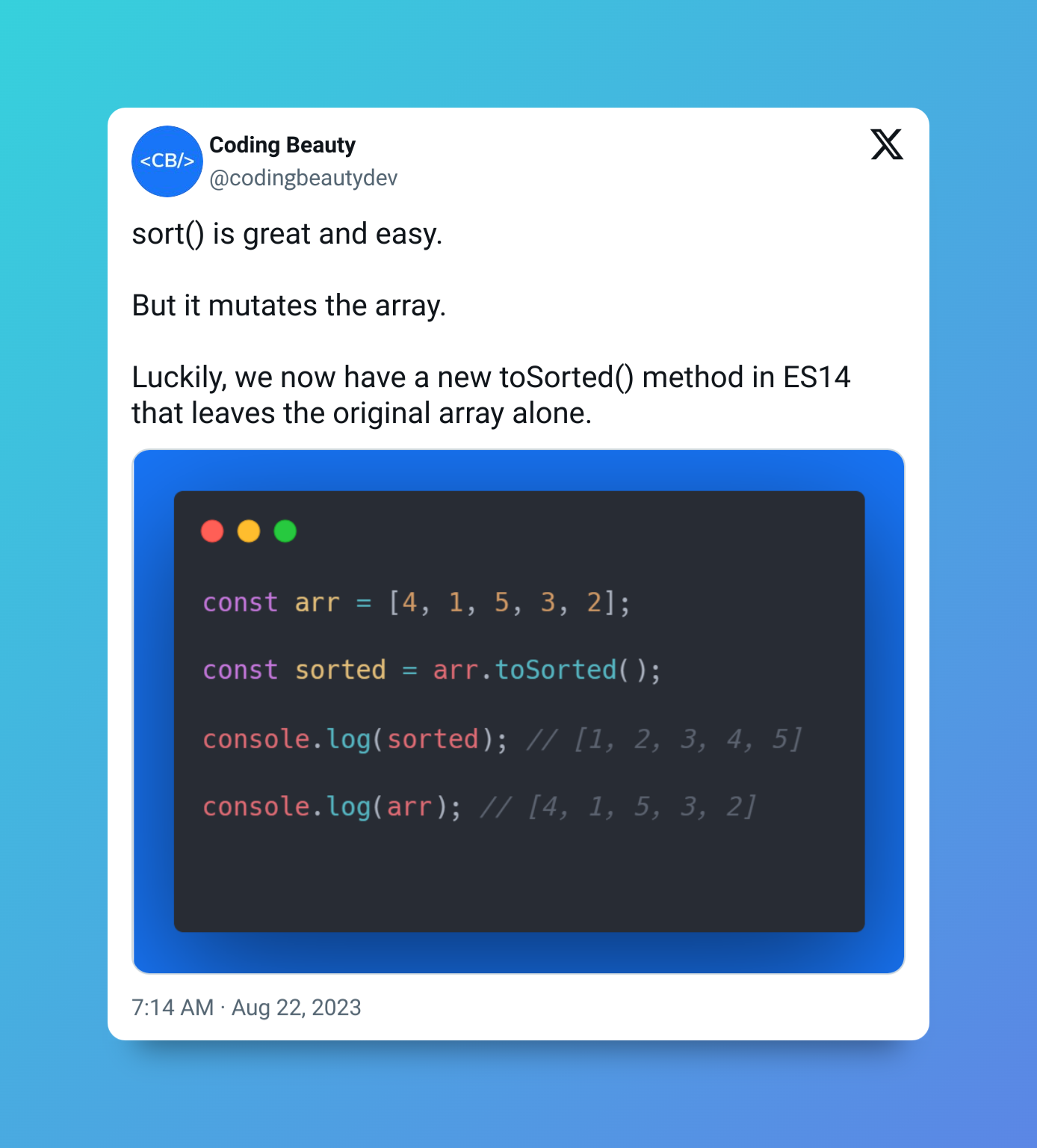# How to quickly get the union of two arrays in JavaScript

To get the union of two arrays in JavaScript, merge the arrays, create a new `Set` object from the merged result to remove the duplicates, then convert it back to an array, i.e., `Array.from(new Set([...arr1, ...arr2]))`.

For example:

JavaScript
``````function getUnion(arr1, arr2) {
return Array.from(new Set([...arr1, ...arr2]));
}

const array1 = [1, 2, 3, 4, 5];
const array2 = [4, 5, 6, 7, 8];

const union = getUnion(array1, array2); // [1, 2, 3, 4, 5, 6, 7, 8]
console.log(union);``````## `Array.from()` method

The `Array from()` method converts `Set`s and other iterable objects to arrays.

JavaScript
``````const set = new Set(['Paul', 'John', 'George', 'Ringo']);
const arr = Array.from(exampleSet);

console.log(arr);  // ['Paul', 'John', 'George', 'Ringo']

const map = new Map();
map.set('Bob', 'Marley');
map.set('Mick', 'Jagger');

let arr2 = Array.from(map);

console.log(exampleArray2);  // [ ['Bob', 'Marley'], ['Mick', 'Jagger'] ]``````

## `Set()` constructor

We create these sets with the `Set` constructor, which can only contain unique values, so it removes any possible duplicates that may be in the array it receives.

JavaScript
``````const set = new Set([1, 1, 2, 3, 3, 4]);
console.log(Array.from(set)); // [1, 2, 3, 4]``````

## `Spread` syntax (`...`)

To pass this array to the `Set()` constructor, we use the spread syntax (`...`) to merge the two arrays together. The spread syntax unpacks the value of each array into another array:

JavaScript
``````const array1 = [10, 20, 30];
const array2 = [40, 50, 60];

const mergedArray = [...array1, ...array2];

console.log(mergedArray);``````

## Use `Array concat()` to merge arrays for `Set()`

We could use the `Array concat()` method in place of the spread syntax:

JavaScript
``````function getUnion(arr1, arr2) {
return Array.from(new Set(arr1.concat(arr2)));
}

const array1 = [1, 2, 3, 4, 5];
const array2 = [4, 5, 6, 7, 8];

const union = getUnion(array1, array2); // [1, 2, 3, 4, 5, 6, 7, 8]
console.log(union);
``````

## Use `Array from()` to convert `Set` back to array

And we could use this spread syntax to replace the `Array from()`, although this may make the code a bit less readable:

JavaScript
``````function getUnion(arr1, arr2) {
return [...new Set([...arr1, ...arr2])];
}

const array1 = [1, 2, 3, 4, 5];
const array2 = [4, 5, 6, 7, 8];

const union = getUnion(array1, array2); // [1, 2, 3, 4, 5, 6, 7, 8]
console.log(union);
``````

We can use the same principles to easily get the union of two sets in JavaScript.

## Get union of two sets in JavaScript

To get the union of two sets in JavaScript, convert the sets to arrays and merge them, then create a `Set` from the results of the merge.

For example:

JavaScript
``````function getUnion(set1, set2) {
return new Set([...set1, ...set2]);
}

const set1 = new Set(['a', 'b', 'c', 'd']);
const set2 = new Set(['c', 'd', 'e', 'f']);

const union = getUnion(set1, set2);

console.log(union); // Set(6) { 'a', 'b', 'c', 'd', 'e', 'f' }``````

## Key takeaways

• To get the union of two arrays in JavaScript, create a new `Set` object from the merge of the two arrays, then convert this `Set` object to an array again, i.e., `Array.from(new Set(arr1.concat(arr2)))`.
• We can do a similar thing to get the union of two sets, i.e., `new Set([...set1, ...set2])`.

## 11 Amazing New JavaScript Features in ES13

This guide will bring you up to speed with all the latest features added in ECMAScript 13. These powerful new features will modernize your JavaScript with shorter and more expressive code.